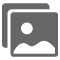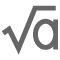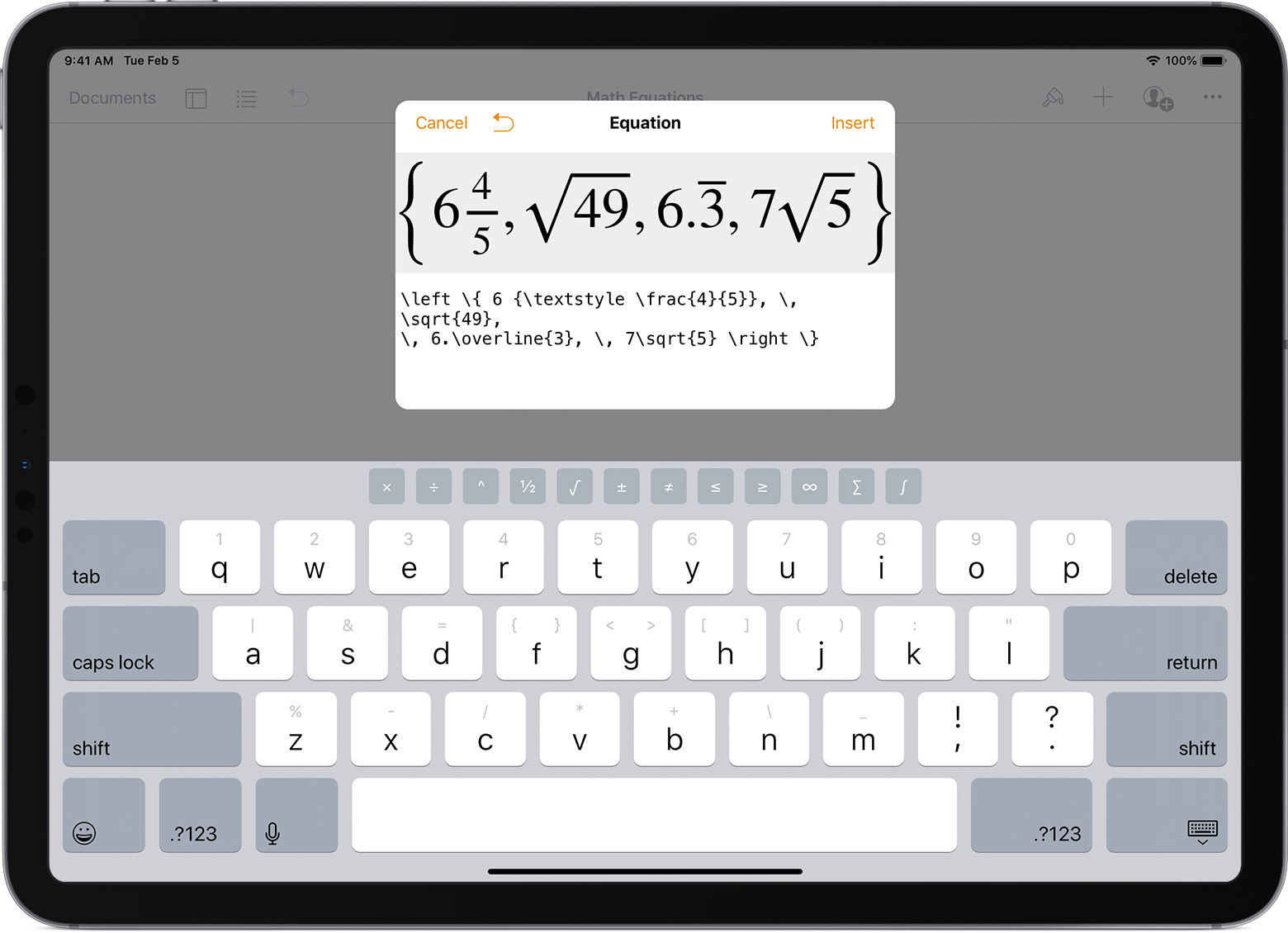# Add mathematical equations to your document in Pages, Numbers, and Keynote

You can include mathematical expressions and equations in your Pages, Numbers, or Keynote document when you use LaTeX commands or MathML elements.

## Add an equation to your Pages, Numbers, or Keynote document

1. Tap or click where you want to add the equation, then follow the steps for your device:
• On your iPhone, iPad, or iPod touch, tap Insert, tap Media, then tap Equation• On your Mac, from the menu bar, choose Insert > Equation.
2. Enter an equation using LaTeX commands or MathML elements.1 On iPhone and iPad, you can also use the shortcut symbols above the keyboard. When you finish typing, a preview of the equation appears.23. Click or tap Insert.3## Use MathType

You can also use MathType with Pages, Numbers, and Keynote for Mac if you have MathType 6.7d (or later) installed. The first time you insert an equation, select Use MathType, or turn it on in Preferences:

1. Open Pages, Numbers, or Keynote.
2. Choose [Application] > Preferences.
3. Select the "Insert and edit equations with MathType" checkbox.## Learn more

Learn which LaTeX commands and MathML elements you can use with Pages, Numbers, and Keynote.

1. To make equation authoring easier, the equation editor is in math mode by default, so it isn't necessary to add math mode commands to your equations.

2. If the preview is empty or displays an error, check your equation to make sure it’s correct.

3. If an equation is by itself on a line of text, the equation centers based on the equals sign. To center based on the middle of the equation, add a space before or after the equation.

Published Date: